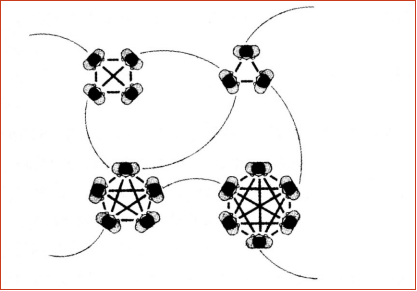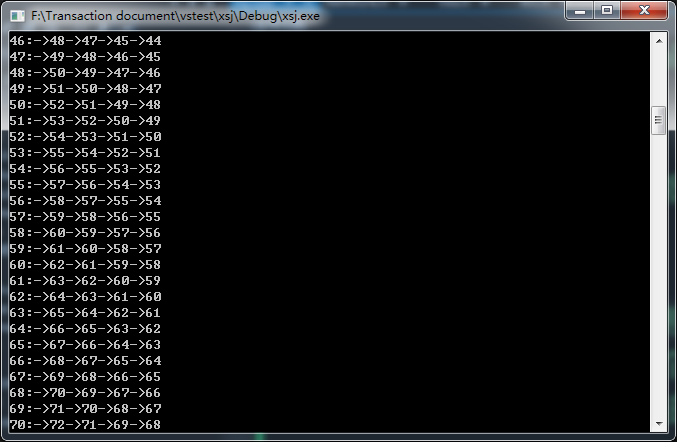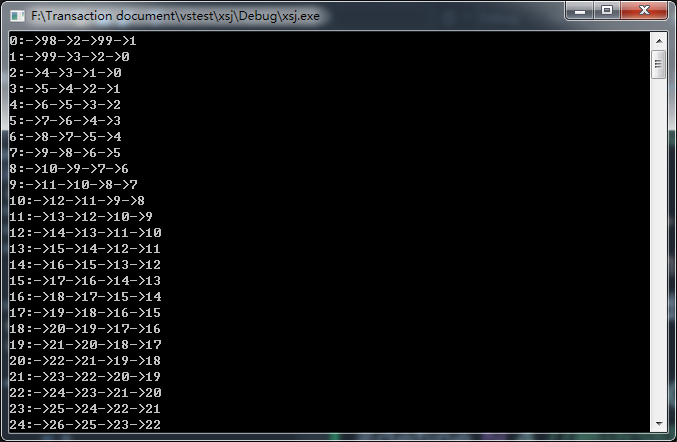# 【复杂网络学习笔记】1：基本知识和小世界网络初步建立①平均路径长：网络中所有节点对的路径长度的平均值。

②聚类系数：假设某个节点有k条边，则这k条边连接的节点（k个）之间最多可能存在的边的条数为k(k-1)/2，用实际存在的边数除以最多可能存在的边数得到的分数值，定义为这个节点的聚类系数。聚类系数是网络的局部特征，反映了相邻两个人之间朋友圈子的重合度，即该节点的朋友之间也是朋友的程度。

①形成N最近邻网络：考虑一个含有N个点的最近邻耦合网络，它们围成一个环，其中每个结点都与其左右相邻的各k/2个结点相连(k为偶数)。

②随机化重连：以概率p随机地重新连接网络中的每个边。即：将边的一个端点保持不变，而另一个端点取为网络中随机选择的一个结点。规定，任意两个不同的结点之间至多有一条边，且每个结点都不能有边与自身相连。

*通用异常类Error.h

#ifndef ERROR_H
#define ERROR_H
#include<iostream>
using namespace std;
//通用异常类
class Error
{
private:
char message;//异常信息
public:
Error(const char *mes = "一般性异常!"); //构造函数
~Error(void) {}; //析构函数
void Show() const; //显示异常信息
};

Error::Error(const char *mes)
{
strcpy_s(message,100, mes); //复制异常信息
}

void Error::Show()const
{
cout << message << endl; //显示异常信息
}
#endif

*无向图邻接网的边节点类Arc.h

#ifndef NULL
#define NULL (void *)0
#endif

#ifndef ARC_H
#define ARC_H

//边节点类
struct Arc
{
int iNum; //弧的另一端的节点的序号
Arc* pNext; //指向下一边节点的指针

Arc(int n, Arc* next); //构造序号为n，下一节点指向next的边节点
};
Arc::Arc(int n,Arc* next)
{
iNum = n;
pNext = next;
}
#endif

*无向图邻接表的顶点节点类Vex.h

#ifndef NULL
#define NULL (void *)0
#endif

#ifndef VEX_H
#define VEX_H
#include "arc.h"
struct Vex
{
Arc* pFirst; //指向边节点表
Vex();//空构造
};
Vex::Vex()
{
pFirst = NULL;
}
#endif

*邻接网络(小世界模型)类NetWork.h

#ifndef NULL
#define NULL (void *)0
#endif

#ifndef NETWORK_H
#define NETWORK_H
#include "vex.h"
#include "error.h"

//邻接网络(小世界模型)类
struct NetWork
{
int vexNum; //顶点的数目
Vex* vexTable; //顶点表

NetWork(int v); //构造有v个顶点的空表
void Clear(); //清空边节点
void BuildVex(int a, int b); //构造从a到b的边
void Step1(int n); //清空全表，然后形成n最近邻网络
void Step2(int r); //以概率r%进行随机化重连
void Show(); //简易的显示该网络
};

NetWork::NetWork(int v) //构造有v个顶点的空表
{
vexTable = new Vex[vexNum=v];
}

void NetWork::Clear() //清空边节点
{
Arc* p;
for (int i = 0; i < vexNum; i++)
{
p = vexTable[i].pFirst; //从第一个边节点开始
while (p != NULL)
{
vexTable[i].pFirst = p->pNext; //first域连到p后去
delete p; //删掉p
p = vexTable[i].pFirst; //再次指向first的节点
}
}
}

void NetWork::BuildVex(int a, int b) //建立从a到b的边
{
if (a < 0 || b < 0 || a >= vexNum || b >= vexNum)
throw Error("数组越界");
if (a == b)
throw Error("不能自身成环");
Arc* p;
bool k = 0; //k=1时表示从a到b有边
p = vexTable[a].pFirst; //不妨看a->b有没有，从第一个边节点开始
while (p != NULL) //检索是否已经有边
{
if (p->iNum == b) //检索到，做标记并跳出
{
k = 1;
break;
}
p = p->pNext; //没检索到，继续找
}
if (k == 0) //如果没有边，建立之
{
vexTable[a].pFirst = new Arc(b, vexTable[a].pFirst); //a能找到b同时
vexTable[b].pFirst = new Arc(a, vexTable[b].pFirst); //b也要能找到a
}
}

void NetWork::Step1(int n) //清空全表，然后形成n最近邻网络
{
if (n > vexNum - 1)
throw Error("n超限");
if (n % 2 != 0)
throw Error("n最近邻网络中n应为偶数");
Clear(); //清空
for (int i = 0; i < vexNum; i++) //对每个节点i
{
for (int j = 1; j <= n / 2; j++) //建立其n最近邻关系
{
BuildVex(i, (i + j) % vexNum); //向后第j个
BuildVex(i, (i - j + vexNum) % vexNum); //向前第j个
}
}
}

void NetWork::Step2(int r) //以概率r%进行随机化重连
{
if (r<0 || r>100)
throw Error("随机化重连的概率不合法");
int a, b; //辅助游标
Arc* p; //辅助指针
for (a = 0; a < vexNum; a++) //对于每个节点
{
p = vexTable[a].pFirst; //从第一条边开始
while (p != NULL) //对于每条边
{
if (rand() % 100 < r) //生成0~99的伪随机数，它小于r的概率应为r%
{
//这里是随机化重连过程
//删除a->旧点和旧点->a的关系
//随机地找一个之前和a无连接的新点
//增加a->新点和新点->a的关系
}
}
}
}

void NetWork::Show() //显示
{
Arc* p; //辅助指针
for (int i = 0; i < vexNum; i++)
{
cout << i << ":";
p = vexTable[i].pFirst; //从第一条边开始
while (p != NULL) //对于每条边
{
cout <<"->"<< p->iNum;
p = p->pNext;
}
cout << endl;
}
}
#endif

*程序入口xsj.cpp

//#pragma warning(disable:4996) //忽略strcpy()函数不安全的warnning
#include "stdafx.h"
#include <iostream>
#include "NetWork.h" //邻接网络(小世界模型)类
using namespace std;

#define VEXNUM 100 //测试的节点个数
#define N 4 //最近邻网络个数
#define R 60 //随机化重连的概率*100

int _tmain(int argc, _TCHAR* argv[])
{
try{
NetWork *pNet = new NetWork(VEXNUM); //建立VEXNUM个节点的空邻接网络
pNet->Step1(N); //形成N最近邻网络
//pNet->Step2(R); //以概率R%随机化重连
pNet->Show(); //显示看一下
system("pause");
return 0;
}
catch (Error er)
{
er.Show();
}
}